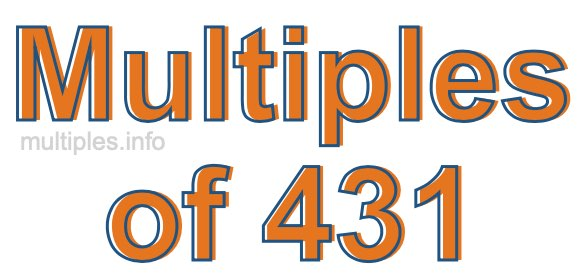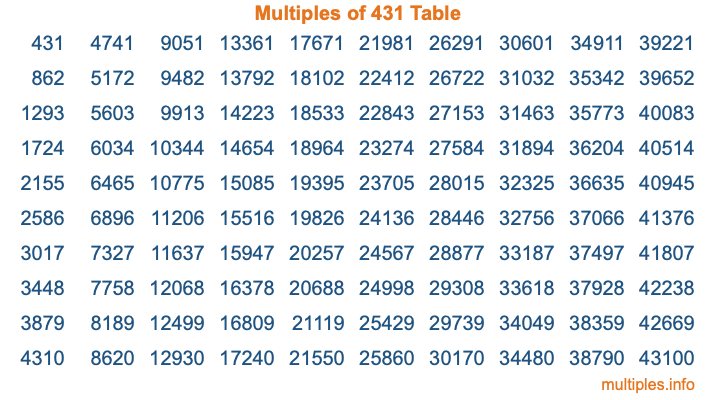Multiples of 431Welcome to the Multiples of 431 page. Here we will first teach you everything you will ever need to know about the multiples of 431, and then give you a study guide summary of everything we taught you to make sure you remember it all. Use this page to look up facts and learn information about the multiples of 431. This page will make you a multiples of four hundred thirty-one expert!

Definition of Multiples of 431
Multiples of 431 are all the numbers that when divided by 431 equal an integer. Each of the multiples of 431 are called a multiple. A multiple of 431 is created by multiplying 431 by an integer.

Therefore, to create a list of multiples of 431, you start with 1 multiplied by 431, then 2 multiplied by 431, then 3 multiplied by 431, and so on for as long as you want. Thus, the list of the first five multiples of 431 is 431, 862, 1293, 1724, and 2155. To see a larger list of multiples of 431, see the printable image of Multiples of 431 further down on this page. We also have a category where you can choose any nth multiple of 431.

Multiples of 431 Checker
The Multiples of 431 Checker below checks to see if any number of your choice is a multiple of 431. In other words, it checks to see if there is any number (integer) that when multiplied by 431 will equal your number. To do that, we divide your number by 431. If the the quotient is an integer, then your number is a multiple of 431.

Is  a multiple of 431?

Least Common Multiple of 431 and ...
A Least Common Multiple (LCM) is the lowest multiple that two or more numbers have in common. This is also called the smallest common multiple or lowest common multiple and is useful to know when you are adding our subtracting fractions. Enter one or more numbers below (431 is already entered) to find the LCM.

Check out our LCM Calculator if you need more details about the Least Common Multiple or if you need the LCM for different numbers for adding and subtraction fractions.

nth Multiple of 431
As we stated above, 431 is the first multiple of 431, 862 is the second multiple of 431, 1293 is the third multiple of 431, and so on. Enter a number below to find the nth multiple of 431.

th multiple of 431

Multiples of 431 vs Factors of 431
431 is a multiple of 431 and a factor of 431, but that is where the similarities end. All postive multiples of 431 are 431 or greater than 431. All positive factors of 431 are 431 or less than 431.

Below is the beginning list of multiples of 431 and the factors of 431 so you can compare:

Multiples of 431: 431, 862, 1293, 1724, 2155, etc.

Factors of 431: 1, 431

As you can see, the multiples of 431 are all the numbers that you can divide by 431 to get a whole number. The factors of 431, on the other hand, are all the whole numbers that you can multiply by another whole number to get 431.

It's also interesting to note that if a number (x) is a factor of 431, then 431 will also be a multiple of that number (x).

Multiples of 431 vs Divisors of 431
The divisors of 431 are all the integers that 431 can be divided by evenly. Below is a list of the divisors of 431.

Divisors of 431: 1, 431

The interesting thing to note here is that if you take any multiple of 431 and divide it by a divisor of 431, you will see that the quotient is an integer.

Multiples of 431 Table
Below is an image of the first 100 multiples of 431 in a table. The table is in chronological order, column by column. The first column has the first ten multiples of 431, the second column has the next ten multiples of 431, and so on.The Multiples of 431 Table is also referred to as the 431 Times Table or Times Table of 431. You are welcome to print out our table for your studies.

Negative Multiples of 431
Although not often discussed or needed in math, it is worth mentioning that you can make a list of negative multiples of 431 by multiplying 431 by -1, then by -2, then by -3, and so on, to get the following list of negative multiples of 431:

-431, -862, -1293, -1724, -2155, etc.

Multiples of 431 Summary
Below is a summary of important Multiples of 431 facts that we have discussed on this page. To retain the knowledge on this page, we recommend that you read through the summary and explain to yourself or a study partner why they hold true.

There are an infinite number of multiples of 431.

A multiple of 431 divided by 431 will equal a whole number.

431 divided by a factor of 431 equals a divisor of 431.

The nth multiple of 431 is n times 431.

The largest factor of 431 is equal to the first positive multiple of 431.

431 is a multiple of every factor of 431.

431 is a multiple of 431.

A multiple of 431 divided by a divisor of 431 equals an integer.

431 divided by a divisor of 431 equals a factor of 431.

Any integer times 431 will equal a multiple of 431.

Multiples of a Number
Here you can get the multiples of another number, all with the same attention to detail as we did for multiples of 431 on this page.

Multiples of
Multiples of 432
Did you find our page about multiples of four hundred thirty-one educational? Do you want more knowledge? Check out the multiples of the next number on our list!